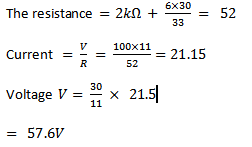Home PHYSICS TOPIC 2: CURRENT ELECTRICITY | PHYSICS FORM 6

# TOPIC 2: CURRENT ELECTRICITY | PHYSICS FORM 6

67
0## TOPIC 2: CURRENT ELECTRICITY | PHYSICS FORM 6

An electric current is set up when a net charge Q passes through any section of the conductor in time t.

The charge assumed to be constant: i.e​​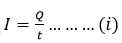If the value of the rate flow of charge is not constant the current varies with time and is given by the equation.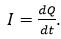#### VELOCITY OF CURRENT CARRIES OR DRIFT VELOCITY (V).

The conduction of electricity in metal is due to free electrons. Free electron have the thermal energy and wonder randomly through the metal from atom to atom and hence collide.

The amplitude of vibration are then increased and the temperature of the metal rise.

On the average the electron drift in the direction of the drift in the mean velocity called drift velocity

Consider a metal wire of cross sectional area A and of length L.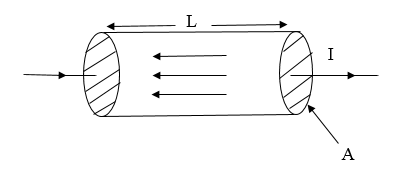Let “n” be the number of electrons per unit volume and “e” is the charge of an electron

The volume occupied by electron is AL

The total number of electrons is nAL

The total charge (Q) in the wire is nALe…….. (1)
​​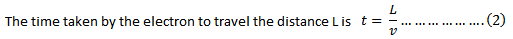If I is the current flowing through this wire then,​​
I = Q/t ———————(3)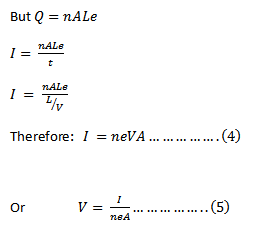From equation  5

​​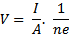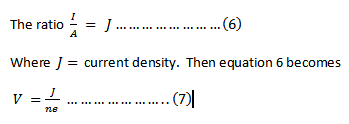Examples​​

•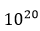Electrons each having a charge passing through point x towards a point y in 0.1 sec. What is the current and its direction?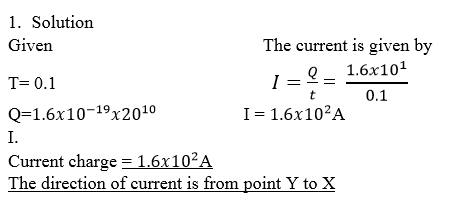2. A conductor with a cross section of​​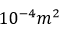and electric current of 1.2A. If the number of electrons be​​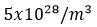calculate the electron velocity.(Take charge of an electron=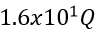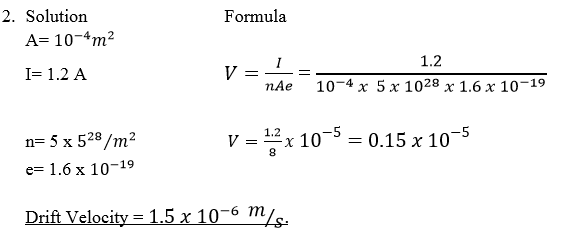Q3 A uniform copper wire of diameter 0.051mm carries a current 10A. what is the drift velocity of electrons in the wire, assuming the charge of electrons is

1.6 X 10-19​​ C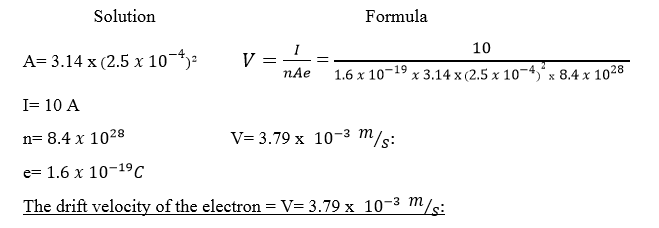#### RESISTANCE AND RESISTIVITY:

Ohm had shown that by using wires of different lengths and diameters.

Resistance of a wire R is proportional to its length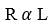and inversely proportional to its cross section area. A i.e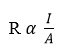From (1) and (2) we have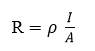Where    is the constant for the material of the wire and it is called. Resistivity.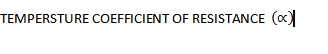The resistance of pure metal increases with temperature.

​​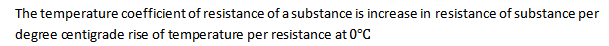​​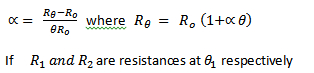​​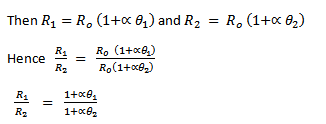Example

A copper coil has a resistance of​​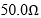at​​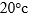what its resistance at​​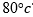?

Take the temperature coefficient of copper is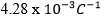Solution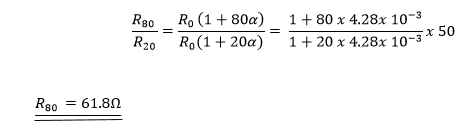HEAT AND ELECTRICAL POWER

Consider an electrical device k and let potential difference between A and B be V.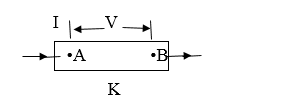If Q is the charge passing through the ends in a time t then Q = It……….. (1)

Total energy given out by the conductor is W = VQ ………………(2)

Substitute eg (1) into eq  (2) we get

W= VIt …… (3)

but workdone = electrical heat developed (H)

hence W= H =VIt …………(4)

Now V= IR so the eqn (4) becomes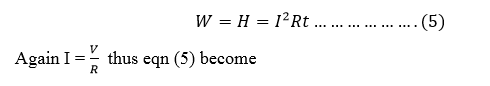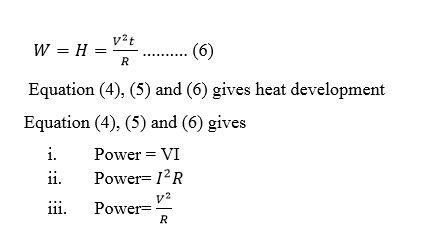KIRCHHOFF’S LAWS

Some circuits cannot be broken down into set of series and parallel combination of conductors. It is then necessary to make use of generalized form of circuits laws already considered. These laws are known as Kirchhoff’s law

Kirchhoff’s first law:

The algebraic sum of current at any junction in the net work is zero

i.e. ∑ I = 0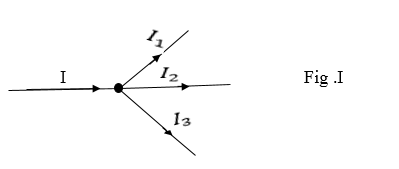A current is given a positive sign if it flows towards a junction and negative sign if it flows away from the junction.

From figure I above we have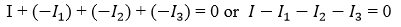Kirchhoff’s second law:

The algebraic sum of emf’s around the loop is equal to the algebraic sum of all potential differences in that circuit i.e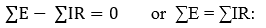RULES FOR FINDING POTENTIAL DIFFERENCE(pd) IN A LOOP

If the resistance is traversed in the direction of the current,the chage in potential is -IR in the opposite direction is +IR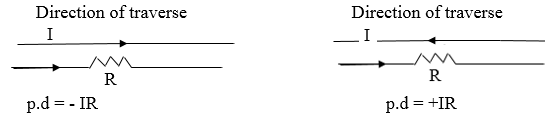If a set of emf is traversed in the direction of emf the change in potential is +ve(+E) in the opposite direction it is(-E)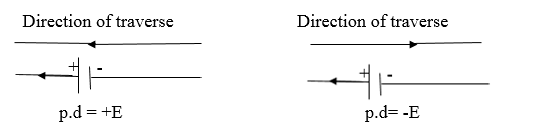The choice of the direction of the traverse depend on yourself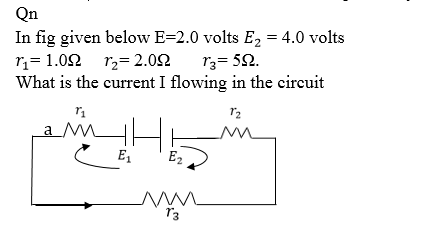Solution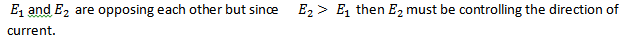Applying Kirchhoff’s laws in the clockwise direction we have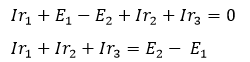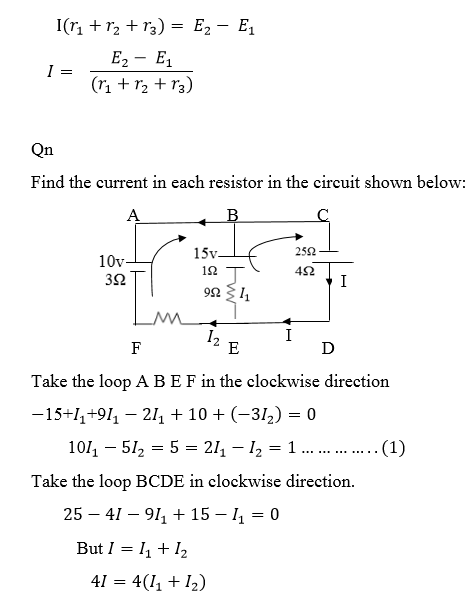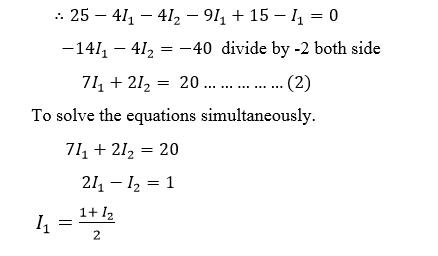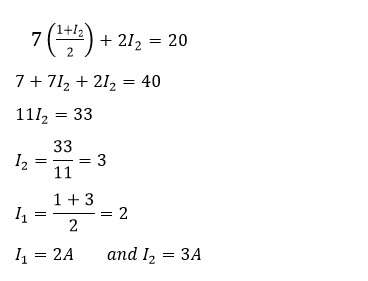Qn.

Determine the magnitude of​​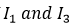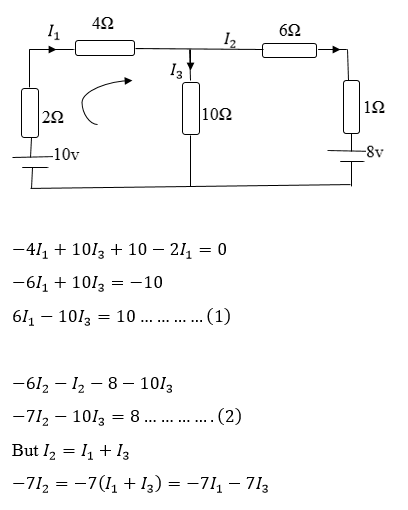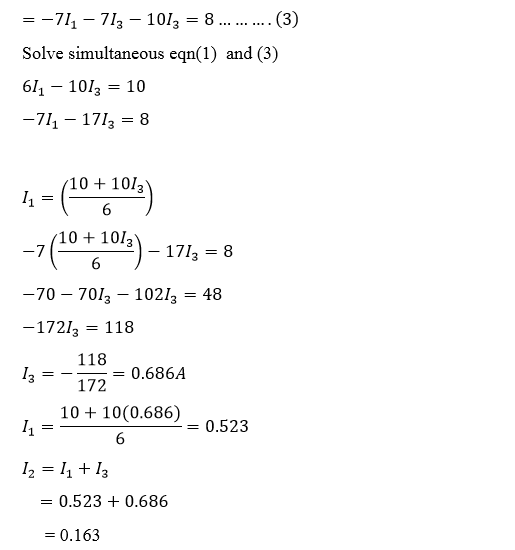The minus sign tells that the current is in of the site direction (from the one assumed)

Qn.
On the basis of Kirchhoff’s laws determine the numerical values for the current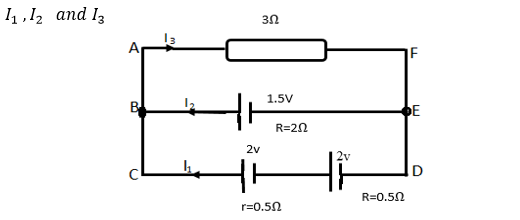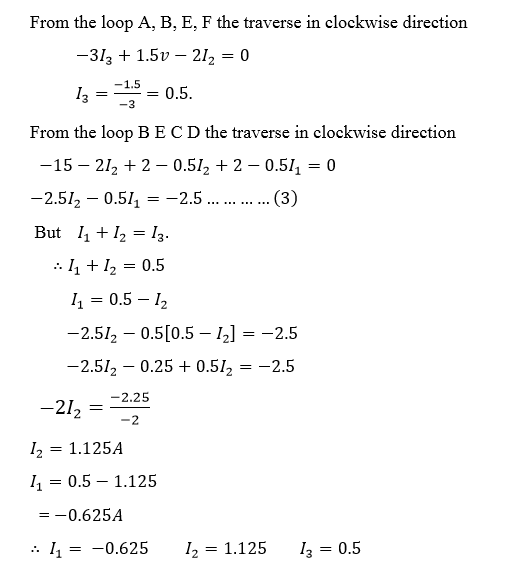Take the loop B E D C in clockwise direction.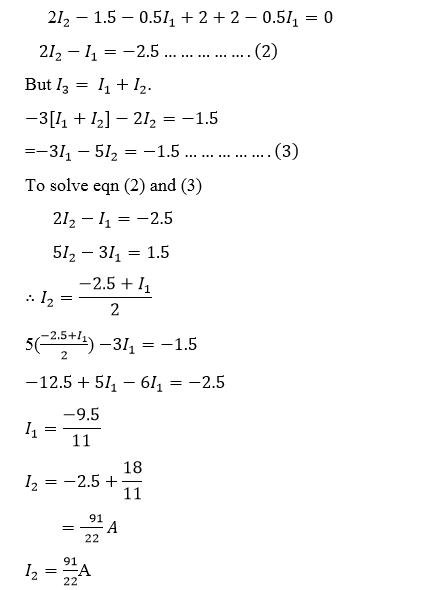POTENTIAL DIVIDER

The resistance box in series are often used to provide known fraction of a given potential difference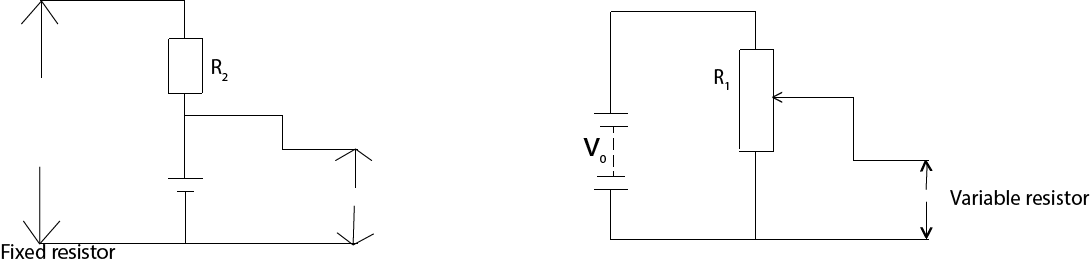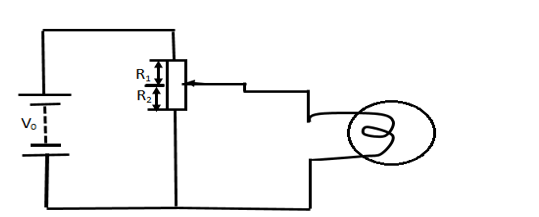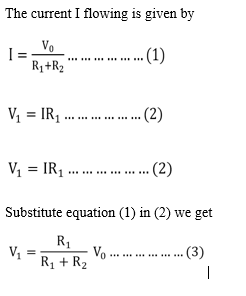A resistor with a sliding contact can similarly be used as shown in figure (ii) to provide continuous variable potential difference from zero to a full supply by value V0.

This is a convenient way of controlling the voltage applied to a load such as lump. The resistor of the load R3  ​​ however acts  in parallel with resistor R1​​ hence equation (1) is no longer true.

Qn.

What is the final potential difference between A and B in the direction circuit below (I) in the circuit as shown (II) if an additional 5000Ω resistor where connected from A to B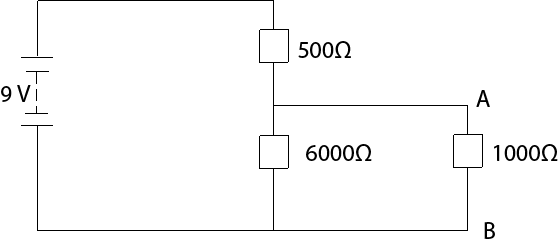Solution

(i)  Final p.d between​​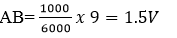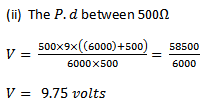​​

#### OHM’S LAW FOR COMPLETE CIRCUIT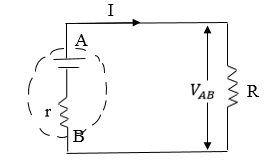There exist certain device such as battery and electronic generators which are able to maintain a potential balance between two points to which they are attached to devices are called a set of electromotive force put the normally have some internal resistances. When the current flow through the battery setsup  a p.d ​​ Ir​​ across the internal resistance. The resistor of resistance  R which is connected to the battery is called the load.

#### OUT PUT AND AN EFFICIENCY

​​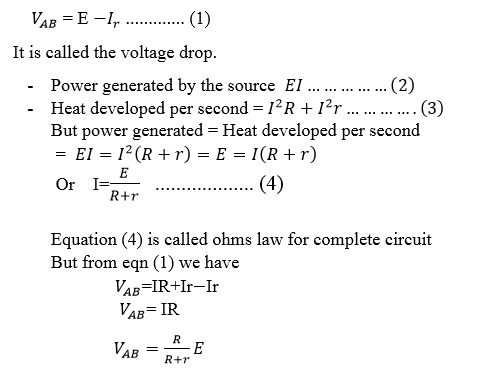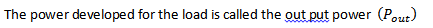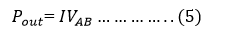power generated by the source the current​​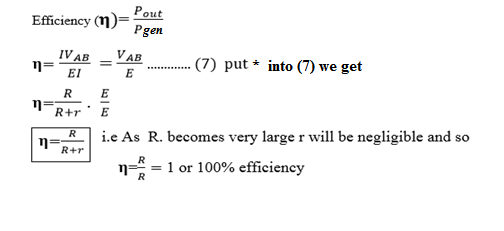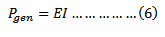#### WHEATSTONE BRIDGE:

– Wheat stone bridge is a circuit that enable resistance to be measured accurately:

Four resistors P, Q , R and S are joined as shown in the figure above

If P is the known resistor Q must be known as well as R and S or their ratio one or more of Q, R and S are adjusted until there is no deflection on G, the bridge is then said to be balanced.

At balance point no current through G so p.d across BD=0 and p.d across AB=p.d across AD. Also current through R = current through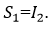​​ Current through p=current through​​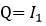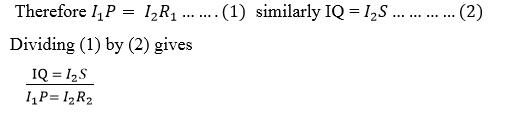​​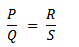​​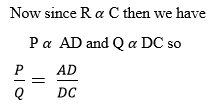Qn.

A wire of length 1.1 meter and Radius​​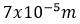is connected across the right gap of the metre bridge when a resistance of 45 Ω is introduced in the left gap from a resistance box connected across it. The balance point is obtained 0.6m from left side. Calculate the specific resistance (resistivity) of the material of the wire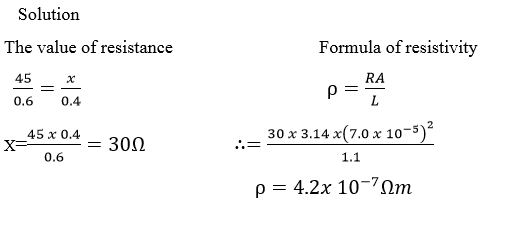Qn.

A​​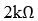and​​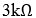resistor are connected in series combination is connected across a 100v supply of negligible internal resistance as shown in the figure below:

(i) What is the output voltage​​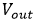of the load resistance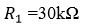(ii) What current resistance through the load resistor​​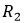in (i) above.

I=21.15.

Solution